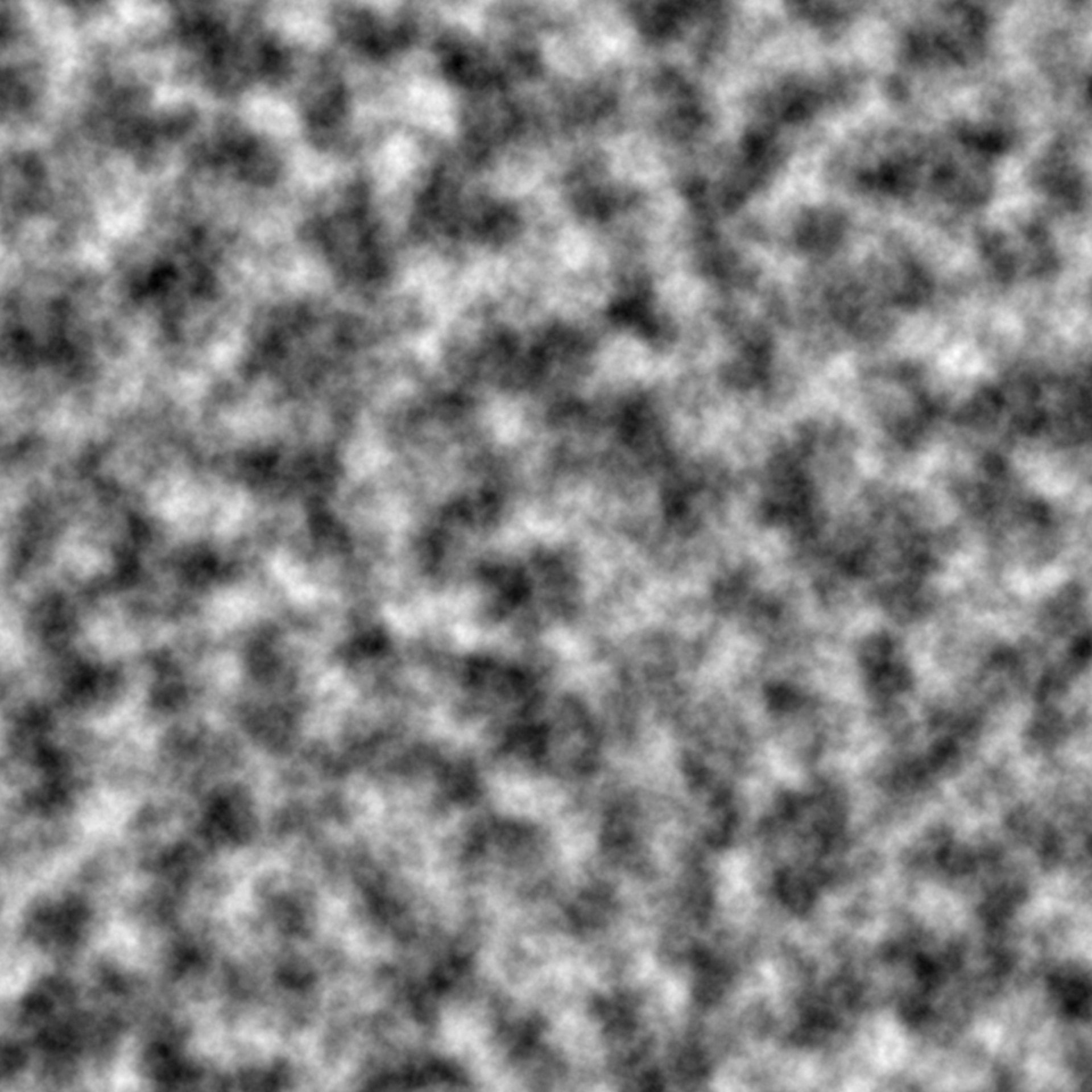This is the archetypal fractal used when generating perlin noise. It works simply by adding successive values together to create a final value. As the succesive values are often calculated at increasing frequencies and the strength is often decreasing, it will create the impression of ever-smaller details as you zoom in. This function is intended to be used in conjunction with fracture()

fbm(base, new, strength, ...)

## Arguments

base The prior values to modify The new values to modify base with A value to modify new with before applying it to base ignored

Other Fractal functions: billow(), clamped(), ridged()
grid <- long_grid(seq(1, 10, length.out = 1000), seq(1, 10, length.out = 1000))
grid$simplex <- fracture(gen_simplex, fbm, octaves = 8, x = grid$x, y = grid\$y)